Protein binding & undfolding – a four-state model¶

In this notebook we will look into a the kinetics of a model system describing competing protein folding, aggregation and ligand binding. Using ChemPy we can define thermodynamic and kinetic parameters, and obtain a representation of a system of ODEs which may be integrated efficiently. Since we use SymPy we can also generate publication quality latex-expressions of our mathematical model directly from our source code. No need to write the equations multiple times in Python/Latex (or even C++ if the integration is to be performed a large number of times such as during parameter estimation).

First we will perform our imports:

In :
import logging; logger = logging.getLogger('matplotlib'); logger.setLevel(logging.INFO)  # or notebook filled with logging

from collections import OrderedDict, defaultdict
import math
import re
import time
from IPython.display import Image, Latex, display
import matplotlib.pyplot as plt
import sympy
from pyodesys.symbolic import ScaledSys
from pyodesys.native.cvode import NativeCvodeSys
from chempy import Substance, Equilibrium, Reaction, ReactionSystem
from chempy.kinetics.ode import get_odesys
from chempy.kinetics.rates import MassAction
from chempy.printing.tables import UnimolecularTable, BimolecularTable
from chempy.thermodynamics.expressions import EqExpr
from chempy.util.graph import rsys2graph
from chempy.util.pyutil import defaultkeydict
%matplotlib inline

Next we will define our substances. Note how we specify the composition, this will allow ChemPy to raise an error if any of our reactions we enter later would violate mass-conservation. It will also allow us to reduce the number of unknowns in our ODE-system by using the linear invariants from the mass-conservation.

In :
substances = OrderedDict([
('N', Substance('N', composition={'protein': 1}, latex_name='[N]')),
('U', Substance('U', composition={'protein': 1}, latex_name='[U]')),
('A', Substance('A', composition={'protein': 1}, latex_name='[A]')),
('L', Substance('L', composition={'ligand': 1}, latex_name='[L]')),
('NL', Substance('NL', composition={'protein': 1, 'ligand': 1}, latex_name='[NL]')),
])

We will model thermodynamic properties using enthalpy (H), entropy (S) and heat capacity (Cp). Kinetic paramaters (rate constants) are assumed to follow the Eyring equation:

In :
def _gibbs(args, T, R, backend, **kwargs):
H, S, Cp, Tref = args
H2 = H + Cp*(T - Tref)
S2 = S + Cp*backend.log(T/Tref)
return backend.exp(-(H2 - T*S2)/(R*T))

def _eyring(args, T, R, k_B, h, backend, **kwargs):
H, S = args
return k_B/h*T*backend.exp(-(H - T*S)/(R*T))
In :
Gibbs = EqExpr.from_callback(_gibbs, parameter_keys=('temperature', 'R'), argument_names=('H', 'S', 'Cp', 'Tref'))
Eyring = MassAction.from_callback(_eyring, parameter_keys=('temperature', 'R', 'k_B', 'h'), argument_names=('H', 'S'))

Next we define our free parameters:

In :
thermo_dis = Gibbs(unique_keys=('He_dis', 'Se_dis', 'Cp_dis', 'Tref_dis'))
thermo_u = Gibbs(unique_keys=('He_u', 'Se_u', 'Cp_u', 'Tref_u'))  # ([He_u_R, Se_u_R, Cp_u_R, Tref])
kinetics_agg = Eyring(unique_keys=('Ha_agg', 'Sa_agg'))  # EyringMassAction([Ha_agg, Sa_agg])
kinetics_as = Eyring(unique_keys=('Ha_as', 'Sa_as'))
kinetics_f = Eyring(unique_keys=('Ha_f', 'Sa_f'))

We will have two reversible reactions, and one irreversible reaction:

In :
eq_dis = Equilibrium({'NL'}, {'N', 'L'}, thermo_dis, name='ligand-protein dissociation')
eq_u = Equilibrium({'N'}, {'U'}, thermo_u, {'L'}, {'L'}, name='protein unfolding')
r_agg = Reaction({'U'}, {'A'}, kinetics_agg, {'L'}, {'L'}, name='protein aggregation')

We formulate a system of 5 reactions honoring our reversible equilibria and our irreversible reaction:

In :
rsys = ReactionSystem(
eq_dis.as_reactions(kb=kinetics_as, new_name='ligand-protein association') +
eq_u.as_reactions(kb=kinetics_f, new_name='protein folding') +
(r_agg,), substances, name='4-state CETSA system')

We can query the ReactionSystem instance for what substances contain what components:

In :
vecs, comp = rsys.composition_balance_vectors()
names = rsys.substance_names()
dict(zip(comp, [dict(zip(names, v)) for v in vecs]))
Out:
{'ligand': {'N': 0, 'U': 0, 'A': 0, 'L': 1, 'NL': 1},
'protein': {'N': 1, 'U': 1, 'A': 1, 'L': 0, 'NL': 1}}

We can look at our ReactionSystem as a graph if we wish:

In :
rsys2graph(rsys, '4state.png', save='.', include_inactive=False)
Image('4state.png')
Out: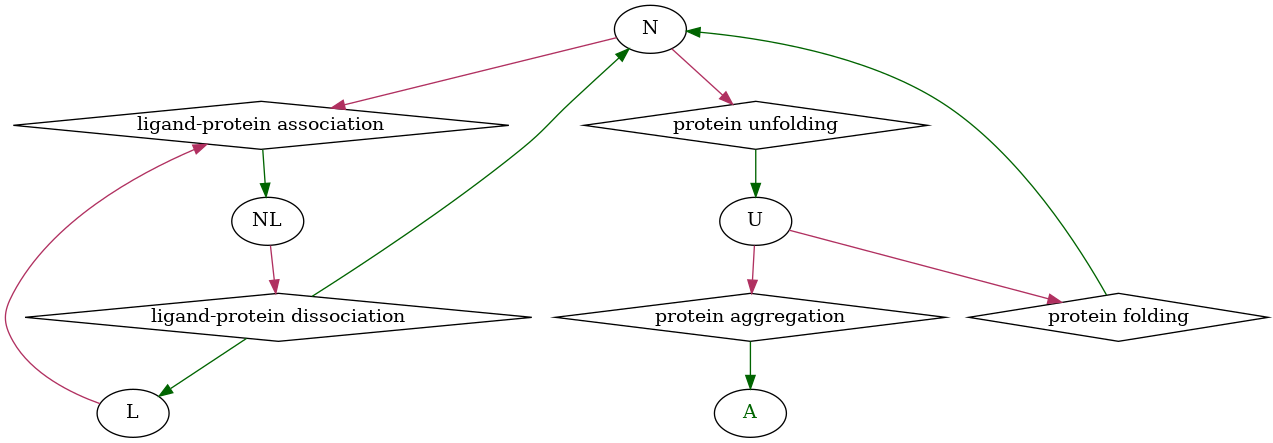...or as a Table if that suits us better (note that "A" ha green highlighting, denoting it's a terminal product)

In :
rsys
Out:
4-state CETSA system
NLL + N MassAction((_MulExpr((_MulExpr((_eyring((), ('Ha_as', 'Sa_as')), _gibbs((), ('He_dis', 'Se_dis', 'Cp_dis', 'Tref_dis')))), Constant((1,)))),)) ligand-protein dissociation
L + NNL MassAction((_eyring((), ('Ha_as', 'Sa_as')),)) ligand-protein association
N + ( L)U + ( L) MassAction((_MulExpr((_MulExpr((_eyring((), ('Ha_f', 'Sa_f')), _gibbs((), ('He_u', 'Se_u', 'Cp_u', 'Tref_u')))), Constant((1,)))),)) protein unfolding
U + ( L)N + ( L) MassAction((_eyring((), ('Ha_f', 'Sa_f')),)) protein folding
U + ( L)A + ( L) MassAction((_eyring((), ('Ha_agg', 'Sa_agg')),)) protein aggregation

Try hovering over the names to have them highlighted (this is particularly useful when working with large reaction sets).

We ca also generate tables representing the unimolecular reactions involing each substance, or the matrix showing the bimolecular reactions:

In :
uni, not_uni = UnimolecularTable.from_ReactionSystem(rsys)
bi, not_bi = BimolecularTable.from_ReactionSystem(rsys)
assert not (not_bi & not_uni), "Only uni- & bi-molecular reactions expected"
In :
uni
Out:
In :
bi
Out:
NUALNL
Nligand-protein association
U-
A--
L---
NL----

Exporting expressions as LaTeX is quite straightforward:

In :
def pretty_replace(s, subs=None):
if subs is None:
subs = {
'Ha_(\w+)': r'\Delta_{\1}H^{\\neq}',
'Sa_(\w+)': r'\Delta_{\1}S^{\\neq}',
'He_(\w+)': r'\Delta_{\1}H^\circ',
'Se_(\w+)': r'\Delta_{\1}S^\circ',
'Cp_(\w+)': r'\Delta_{\1}\,C_p',
'Tref_(\w+)': r'T^{\circ}_{\1}',
}
for pattern, repl in subs.items():
s = re.sub(pattern, repl, s)
return s

def mk_Symbol(key):
if key in substances:
arg = substances[key].latex_name
else:
arg = pretty_replace(key.replace('temperature', 'T'))

return sympy.Symbol(arg)

autosymbols = defaultkeydict(mk_Symbol)
rnames = {}
for rxn in rsys.rxns:
rnames[rxn.name] = rxn.name.replace(' ', '~').replace('-','-')
rate_expr_str = sympy.latex(rxn.rate_expr()(autosymbols, backend=sympy, reaction=rxn))
lstr = r'$r(\mathrm{%s}) = %s$' % (rnames[rxn.name], rate_expr_str)
display(Latex(lstr))
$r(\mathrm{ligand-protein~dissociation}) = \frac{T [NL] k_{B} e^{\frac{T \Delta_{as}S^{\neq} - \Delta_{as}H^{\neq}}{R T}} e^{\frac{T \left(\Delta_{dis}S^\circ + \Delta_{dis}\,C_p \log{\left(\frac{T}{T^{\circ}_{dis}} \right)}\right) - \Delta_{dis}H^\circ - \Delta_{dis}\,C_p \left(T - T^{\circ}_{dis}\right)}{R T}}}{h}$
$r(\mathrm{ligand-protein~association}) = \frac{T [L] [N] k_{B} e^{\frac{T \Delta_{as}S^{\neq} - \Delta_{as}H^{\neq}}{R T}}}{h}$
$r(\mathrm{protein~unfolding}) = \frac{T [N] k_{B} e^{\frac{T \Delta_{f}S^{\neq} - \Delta_{f}H^{\neq}}{R T}} e^{\frac{T \left(\Delta_{u}S^\circ + \Delta_{u}\,C_p \log{\left(\frac{T}{T^{\circ}_{u}} \right)}\right) - \Delta_{u}H^\circ - \Delta_{u}\,C_p \left(T - T^{\circ}_{u}\right)}{R T}}}{h}$
$r(\mathrm{protein~folding}) = \frac{T [U] k_{B} e^{\frac{T \Delta_{f}S^{\neq} - \Delta_{f}H^{\neq}}{R T}}}{h}$
$r(\mathrm{protein~aggregation}) = \frac{T [U] k_{B} e^{\frac{T \Delta_{agg}S^{\neq} - \Delta_{agg}H^{\neq}}{R T}}}{h}$
In :
ratexs = [autosymbols['r(\mathrm{%s})' % rnames[rxn.name]] for rxn in rsys.rxns]
rates = rsys.rates(autosymbols, backend=sympy, ratexs=ratexs)
for k, v in rates.items():
display(Latex(r'$\frac{[%s]}{dt} = %s$' % (k, sympy.latex(v))))
$\frac{[NL]}{dt} = r(\mathrm{ligand-protein~association}) - r(\mathrm{ligand-protein~dissociation})$
$\frac{[L]}{dt} = - r(\mathrm{ligand-protein~association}) + r(\mathrm{ligand-protein~dissociation})$
$\frac{[N]}{dt} = - r(\mathrm{ligand-protein~association}) + r(\mathrm{ligand-protein~dissociation}) + r(\mathrm{protein~folding}) - r(\mathrm{protein~unfolding})$
$\frac{[U]}{dt} = - r(\mathrm{protein~aggregation}) - r(\mathrm{protein~folding}) + r(\mathrm{protein~unfolding})$
$\frac{[A]}{dt} = r(\mathrm{protein~aggregation})$
In :
default_c0 = defaultdict(float, {'N': 1e-9, 'L': 1e-8})

params = dict(
R=8.314472,  # or N_A & k_B
k_B=1.3806504e-23,
h=6.62606896e-34,  # k_B/h == 2.083664399411865e10 K**-1 * s**-1
He_dis=-45e3,
Se_dis=-400,
Cp_dis=1.78e3,
Tref_dis=298.15,
He_u=60e3,
Cp_u=20.5e3,
Tref_u=298.15,
Ha_agg=106e3,
Sa_agg=70,
Ha_as=4e3,
Sa_as=-10,
Ha_f=90e3,
Sa_f=50,
temperature=50 + 273.15
)

We have the melting temperature $T_m$ as a free parameter, however, the model is expressed in terms of $\Delta_u S ^\circ$ so will need to derive the latter from the former: $$\begin{cases} \Delta G = 0 \\ \Delta G = \Delta H - T_m\Delta_u S \end{cases}$$ $$\begin{cases} \Delta H = \Delta H^\circ + \Delta C_p \left( T_m - T^\circ \right) \\ \Delta S = \Delta S^\circ + \Delta C_p \ln\left( \frac{T_m}{T^\circ} \right) \end{cases}$$ this gives us the following equation: $$\Delta H^\circ + \Delta C_p \left( T_m - T^\circ \right) = T_m \left( \Delta S^\circ + \Delta C_p \ln\left( \frac{T_m}{T^\circ} \right) \right)$$ Solving for $\Delta S^\circ$: $$\Delta S^\circ = T_m^{-1}\left( \Delta H^\circ + \Delta C_p \left( T_m - T^\circ \right) \right) - \Delta C_p \ln\left( \frac{T_m}{T^\circ} \right)$$

In :
def Se0_from_Tm(Tm, token):
dH0, T0, dCp = params['He_'+token], params['Tref_'+token], params['Cp_'+token]
return dH0/Tm + (Tm-T0)*dCp/Tm - dCp*math.log(Tm/T0)
params['Se_u'] = Se0_from_Tm(48.2+273.15, 'u')
params['Se_u']
Out:
130.5683281088168

If we want to see the numerical values for the rate of the individual reactions it is quite easy:

In :
params_c0 = default_c0.copy()
params_c0.update(params)
for rxn in rsys.rxns:
print('%s: %.5g' % (rxn.name, rxn.rate_expr()(params_c0, reaction=rxn)))
ligand-protein dissociation: 0
ligand-protein association: 4.564e-06
protein unfolding: 2.4776e-08
protein folding: 0
protein aggregation: 0

By using pyodesys we can generate a system of ordinary differential equations:

In :
odesys, extra = get_odesys(rsys, include_params=False, SymbolicSys=ScaledSys, dep_scaling=1e9)
len(odesys.exprs)  # how many (symbolic) expressions are there in this representation?
Out:
5

Numerical integration of ODE systems require a guess for the inital step-size. We can derive an upper bound for an "Euler-forward step" from initial concentrations and restrictions on mass-conservation:

In :
h0max = extra['max_euler_step_cb'](0, default_c0, params)
h0max
Out:
0.00021792519480996216

Now let's put our ODE-system to work:

In :
def integrate_and_plot(system, c0=None, first_step=None, t0=0, stiffness=False, nsteps=9000, **kwargs):
if c0 is None:
c0 = default_c0
if first_step is None:
first_step = h0max*1e-11
tend = 3600*24
t_py = time.time()

kwargs['atol'] = kwargs.get('atol', 1e-11)
kwargs['rtol'] = kwargs.get('rtol', 1e-11)
res = system.integrate([t0, tend], c0, params, integrator='cvode', nsteps=nsteps,
first_step=first_step, **kwargs)
t_py = time.time() - t_py
if stiffness:
plt.subplot(1, 2, 1)
_ = system.plot_result(xscale='log', yscale='log')
_ = plt.legend(loc='best')
plt.gca().set_ylim([1e-16, 1e-7])
plt.gca().set_xlim([1e-11, tend])

if stiffness:
if stiffness is True:
stiffness = 0
ratios = odesys.stiffness()
plt.subplot(1, 2, 2)
plt.yscale('linear')
plt.plot(odesys._internal[stiffness:], ratios[stiffness:])
for k in ('time_wall', 'time_cpu'):
print('%s = %.3g' % (k, res[k]), end=', ')
print('time_python = %.3g' % t_py)
return res

_, _, info = integrate_and_plot(odesys)
assert info['internal_yout'].shape == 5
{k: v for k, v in info.items() if not k.startswith('internal')}
time_wall = 0.477, time_cpu = 0.473, time_python = 0.483
Out:
{'njvev': 0,
'nfev': 792,
'n_dls_rhs_evals': 0,
'n_dls_jac_evals': 13,
'n_root_evals': 0,
'n_nonlin_solv_iters': 791,
'n_steps': 718,
'njev': 13,
'n_nonlin_solv_conv_fails': 0,
'n_rhs_evals': 792,
'n_lin_solv_setups': 90,
'n_err_test_fails': 9,
'time_prec': 0.0,
'time_jtimes': 0.0,
'time_wall': 0.477007344,
'time_rhs': 0.46101644300000005,
'time_roots': 0.0,
'time_cpu': 0.472574,
'time_jac': 0.008959799999999999,
'steps': array([], dtype=float64),
'fpes': array([], dtype=int64),
'orders': array([], dtype=int64),
'success': True,
'atol': [1e-11],
'rtol': 1e-11,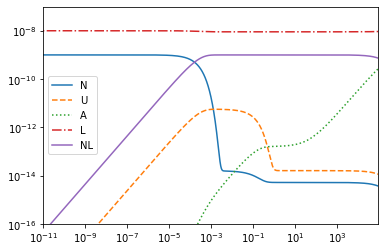pyodesys even allows us to generate C++ code which is compiled to a fast native extension module:

In :
native = NativeCvodeSys.from_other(odesys, first_step_expr=0*odesys.indep)
In :
_, _, info_native = integrate_and_plot(native)
{k: v for k, v in info_native.items() if not k.startswith('internal')}
INFO:pyodesys.native._base:In "/tmp/tmp8v5wjpbg_pycodeexport_pyodesys_NativeCvodeCode", executing:
"/usr/bin/g++ -c -std=c++11 -Wall -Wextra -fPIC -O2 -ffast-math -funroll-loops -fopenmp -o ./odesys_anyode.o -DPYCVODES_NO_KLU=1 -DPYCVODES_NO_LAPACK=0 -DANYODE_NO_LAPACK=0 -I/usr/local/lib/python3.6/dist-packages/numpy/core/include -I/drone/src/github.com/bjodah/chempy/ci_cache/pyusrb/lib/python3.6/site-packages/pyodesys/native/sources -I/drone/src/github.com/bjodah/chempy/ci_cache/pyusrb/lib/python3.6/site-packages/pycvodes/include odesys_anyode.cpp"
INFO:pyodesys.native._base:In "/tmp/tmp8v5wjpbg_pycodeexport_pyodesys_NativeCvodeCode", executing:
"/usr/bin/g++ -pthread -shared -std=c++11 -Wall -Wextra -fPIC -O2 -ffast-math -funroll-loops -fopenmp -o /tmp/tmp8v5wjpbg_pycodeexport_pyodesys_NativeCvodeCode/_cvode_wrapper.cpython-36m-x86_64-linux-gnu.so -DPYCVODES_NO_KLU=1 -DPYCVODES_NO_LAPACK=0 -DANYODE_NO_LAPACK=0 -I/usr/local/lib/python3.6/dist-packages/numpy/core/include -I/drone/src/github.com/bjodah/chempy/ci_cache/pyusrb/lib/python3.6/site-packages/pyodesys/native/sources -I/drone/src/github.com/bjodah/chempy/ci_cache/pyusrb/lib/python3.6/site-packages/pycvodes/include odesys_anyode.o _cvode_wrapper.o -lsundials_nvecserial -lsundials_cvodes -lsundials_sunlinsollapackdense -lsundials_sunlinsollapackband -lblas -llapack -lpython3.6m"
time_wall = 0.00354, time_cpu = 0.00354, time_python = 2.92
Out:
{'n_err_test_fails': 15,
'n_lin_solv_setups': 100,
'n_rhs_evals': 831,
'n_nonlin_solv_conv_fails': 0,
'njev': 13,
'n_steps': 737,
'nfev': 831,
'njvev': 0,
'n_dls_rhs_evals': 0,
'n_dls_jac_evals': 13,
'n_root_evals': 0,
'n_nonlin_solv_iters': 830,
'time_jac': 1.552e-06,
'time_cpu': 0.0035399999999999997,
'time_prec': 0.0,
'time_jtimes': 0.0,
'time_rhs': 0.00011868299999999995,
'time_wall': 0.003538872,
'time_roots': 0.0,
'steps': array([], dtype=float64),
'orders': array([], dtype=int64),
'fpes': array([], dtype=int64),
'root_indices': [],
'success': True}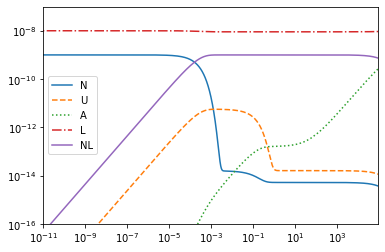Note how much smaller "time_cpu" was here

In :
info['time_wall']/info_native['time_wall']
Out:
134.79078757298936
In :
from chempy.kinetics._native import get_native
In :
native2 = get_native(rsys, odesys, 'cvode')
In :
_, _, info_native2 = integrate_and_plot(native2, first_step=0.0)
{k: v for k, v in info_native2.items() if not k.startswith('internal')}
INFO:pyodesys.native._base:In "/tmp/tmp2rppep09_pycodeexport_pyodesys_NativeCvodeCode", executing:
"/usr/bin/g++ -c -std=c++11 -Wall -Wextra -fPIC -O2 -ffast-math -funroll-loops -fopenmp -o ./odesys_anyode.o -DPYCVODES_NO_KLU=1 -DPYCVODES_NO_LAPACK=0 -DANYODE_NO_LAPACK=0 -I/usr/local/lib/python3.6/dist-packages/numpy/core/include -I/drone/src/github.com/bjodah/chempy/ci_cache/pyusrb/lib/python3.6/site-packages/pyodesys/native/sources -I/drone/src/github.com/bjodah/chempy/ci_cache/pyusrb/lib/python3.6/site-packages/pycvodes/include odesys_anyode.cpp"
INFO:pyodesys.native._base:In "/tmp/tmp2rppep09_pycodeexport_pyodesys_NativeCvodeCode", executing:
"/usr/bin/g++ -pthread -shared -std=c++11 -Wall -Wextra -fPIC -O2 -ffast-math -funroll-loops -fopenmp -o /tmp/tmp2rppep09_pycodeexport_pyodesys_NativeCvodeCode/_cvode_wrapper.cpython-36m-x86_64-linux-gnu.so -DPYCVODES_NO_KLU=1 -DPYCVODES_NO_LAPACK=0 -DANYODE_NO_LAPACK=0 -I/usr/local/lib/python3.6/dist-packages/numpy/core/include -I/drone/src/github.com/bjodah/chempy/ci_cache/pyusrb/lib/python3.6/site-packages/pyodesys/native/sources -I/drone/src/github.com/bjodah/chempy/ci_cache/pyusrb/lib/python3.6/site-packages/pycvodes/include odesys_anyode.o _cvode_wrapper.o -lsundials_nvecserial -lsundials_cvodes -lsundials_sunlinsollapackdense -lsundials_sunlinsollapackband -lblas -llapack -lpython3.6m"
time_wall = 0.00301, time_cpu = 0.003, time_python = 2.79
Out:
{'n_err_test_fails': 10,
'n_lin_solv_setups': 91,
'n_rhs_evals': 809,
'n_nonlin_solv_conv_fails': 0,
'njev': 13,
'n_steps': 733,
'nfev': 810,
'njvev': 0,
'n_dls_rhs_evals': 0,
'n_dls_jac_evals': 13,
'n_root_evals': 0,
'n_nonlin_solv_iters': 808,
'time_jac': 1.4269999999999998e-06,
'time_cpu': 0.0030039999999999997,
'time_prec': 0.0,
'time_jtimes': 0.0,
'time_rhs': 0.00011789000000000009,
'time_wall': 0.003006276,
'time_roots': 0.0,
'steps': array([], dtype=float64),
'orders': array([], dtype=int64),
'fpes': array([], dtype=int64),
'root_indices': [],
'success': True}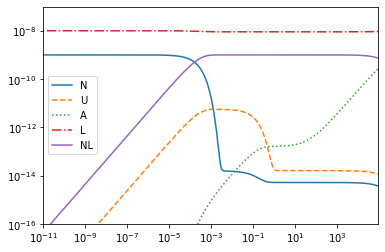We have one complication, due to linear dependencies in our formulation of the system of ODEs our jacobian is singular:

In :
cses, (jac_in_cse,) = odesys.be.cse(odesys.get_jac())
jac_in_cse
Out:
$\displaystyle \left[\begin{matrix}x_{5} - x_{8} & x_{7} & 0 & x_{10} & x_{11}\\x_{8} & - x_{12} - x_{7} & 0 & 0 & 0\\0 & x_{12} & 0 & 0 & 0\\x_{5} & 0 & 0 & x_{10} & x_{11}\\x_{4} & 0 & 0 & x_{9} & - x_{11}\end{matrix}\right]$
In :
odesys.jacobian_singular()
Out:
True

Since implicit methods (which are required for stiff cases often encountered in kinetic modelling) uses the Jacboian (or rather I - γJ) in the modified Newton's method we may get failures during integration (depending on step size and scaling). What we can do is to identify linear dependencies based on composition of the materials and exploit the invariants to reduce the dimensionality of the system of ODEs:

In :
A, comp_names = rsys.composition_balance_vectors()
A, comp_names, list(rsys.substances.keys())
Out:
([[0, 0, 0, 1, 1], [1, 1, 1, 0, 1]],
['ligand', 'protein'],
['N', 'U', 'A', 'L', 'NL'])

That made sense: two different components can give us (up to) two linear invariants.

Let's look what those invariants looks like symbolically:

In :
y0 = {odesys[k]: sympy.Symbol(k+'0') for k in rsys.substances.keys()}
analytic_L_N = extra['linear_dependencies'](['L', 'N'])
analytic_L_N(None, y0, None, sympy)
assert len(analytic_L_N(None, y0, None, sympy)) > 0  # ensure the callback is idempotent
analytic_L_N(None, y0, None, sympy), list(enumerate(odesys.names))
Out:
(OrderedDict([(y_0, A0 + N0 + NL0 + U0 - y_1 - y_2 - y_4),
(y_3, L0 + NL0 - y_4)]),
[(0, 'N'), (1, 'U'), (2, 'A'), (3, 'L'), (4, 'NL')])

one can appreciate that one does not need to enter such expressions manually (at least for larger systems). That is both tedious and error prone.

Let's see how we can use pyodesys to leverage this information on redundancy:

In :
from pyodesys.symbolic import PartiallySolvedSystem
no_invar = dict(linear_invariants=None, linear_invariant_names=None)
psysLN = PartiallySolvedSystem(odesys, analytic_L_N, **no_invar)
print(psysLN.be.cse(psysLN.get_jac()))
psysLN['L'], psysLN.jacobian_singular(), len(psysLN.exprs)
Matrix([[-x3 - x4 + x5, x5, x5], [x4, 0, 0], [x9, x9, -x2*x7*exp(x0*(p_2*(p_7 + p_8*log(p_2/p_9)) - p_6 - p_8*(p_2 - p_9))) - x8*(i_A + i_N + i_U + x6 - y_1 - y_2) + x9]])
Out:
(i_L + i_NL - y_4, False, 3)

above we chose to get rid of 'L' and 'N', but we could also have removed 'A' instead of 'N':

In :
psysLA = PartiallySolvedSystem(odesys, extra['linear_dependencies'](['L', 'A']), **no_invar)
print(psysLA.be.cse(psysLA.get_jac()))
psysLA['L'], psysLA.jacobian_singular()
Matrix([[-x4 - x7, x6, x8 + x9], [x7, -x5*exp(x0*(-p_16 + p_17*p_2)) - x6, 0], [x4, 0, -x8 - x9]])
Out:
(i_L + i_NL - y_4, False)
In :
plt.figure(figsize=(12,4))
plt.subplot(1, 2, 1)
_, _, info_LN = integrate_and_plot(psysLN, first_step=0.0)
assert info_LN['internal_yout'].shape == 3

plt.subplot(1, 2, 2)
_, _, info_LA = integrate_and_plot(psysLA, first_step=0.0)
assert info_LA['internal_yout'].shape == 3

({k: v for k, v in info_LN.items() if not k.startswith('internal')},
{k: v for k, v in info_LA.items() if not k.startswith('internal')})
time_wall = 0.382, time_cpu = 0.38, time_python = 0.385
time_wall = 0.306, time_cpu = 0.307, time_python = 0.307
Out:
({'njvev': 0,
'nfev': 779,
'n_dls_rhs_evals': 0,
'n_dls_jac_evals': 12,
'n_root_evals': 0,
'n_nonlin_solv_iters': 778,
'n_steps': 691,
'njev': 12,
'n_nonlin_solv_conv_fails': 0,
'n_rhs_evals': 779,
'n_lin_solv_setups': 91,
'n_err_test_fails': 12,
'time_prec': 0.0,
'time_jtimes': 0.0,
'time_wall': 0.382441578,
'time_rhs': 0.36535629999999963,
'time_roots': 0.0,
'time_cpu': 0.380247,
'time_jac': 0.009410641999999999,
'steps': array([], dtype=float64),
'fpes': array([], dtype=int64),
'orders': array([], dtype=int64),
'success': True,
'atol': [1e-11],
'rtol': 1e-11,
{'njvev': 0,
'nfev': 860,
'n_dls_rhs_evals': 0,
'n_dls_jac_evals': 13,
'n_root_evals': 0,
'n_nonlin_solv_iters': 859,
'n_steps': 760,
'njev': 13,
'n_nonlin_solv_conv_fails': 0,
'n_rhs_evals': 860,
'n_lin_solv_setups': 98,
'n_err_test_fails': 16,
'time_prec': 0.0,
'time_jtimes': 0.0,
'time_wall': 0.305868383,
'time_rhs': 0.2956507100000002,
'time_roots': 0.0,
'time_cpu': 0.307158,
'time_jac': 0.004656188,
'steps': array([], dtype=float64),
'fpes': array([], dtype=int64),
'orders': array([], dtype=int64),
'success': True,
'atol': [1e-11],
'rtol': 1e-11,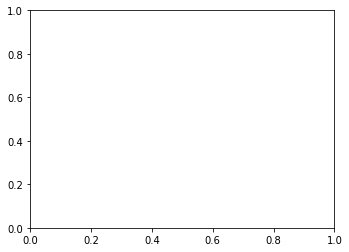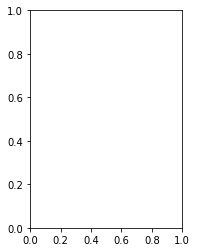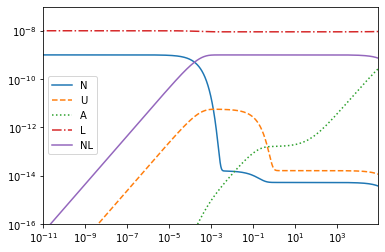We can also have the solver return to use when some precondition is fulfilled, e.g. when the concentraion of 'N' and 'A' are equal:

In :
from pyodesys.symbolic import SymbolicSys
psys_root = SymbolicSys.from_other(psysLN, roots=[psysLN['N'] - psysLN['A']])
In :
psys_root.roots
Out:
[i_A + i_N + i_NL + i_U - y_1 - 2*y_2 - y_4]
In :
psysLN['N']
Out:
$\displaystyle i_{A} + i_{N} + i_{NL} + i_{U} - y_{1} - y_{2} - y_{4}$
In :
psysLN.analytic_exprs
Out:
OrderedDict([(y_0, i_A + i_N + i_NL + i_U - y_1 - y_2 - y_4),
(y_3, i_L + i_NL - y_4)])
In :
psysLN.names
Out:
('N', 'U', 'A', 'L', 'NL')
In :
psysLN.dep
Out:
(y_1, y_2, y_4)
In :
tout1, Cout1, info_root = integrate_and_plot(psys_root, first_step=0.0, return_on_root=True)
print('Time at which concnetrations of N & A are equal: %.4g' % (tout1[-1]))
time_wall = 0.211, time_cpu = 0.211, time_python = 0.213
Time at which concnetrations of N & A are equal: 0.01253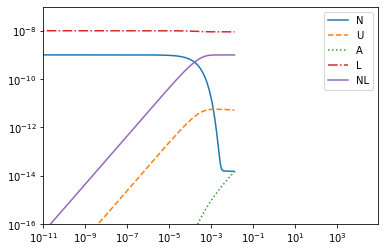From this point in time onwards we could for example choose to continue our integration using another formulation of the ODE-system:

In :
xout2, yout2, info_LA = integrate_and_plot(psysLA, first_step=0.0, t0=tout1[-1], c0=dict(zip(odesys.names, Cout1[-1, :])))
time_wall = 0.124, time_cpu = 0.125, time_python = 0.126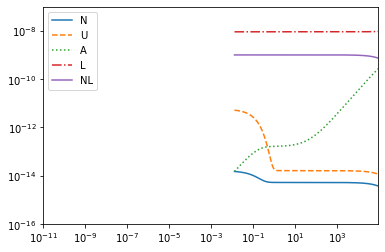Let's compare the total number steps needed for our different approaches:

In :
print('\troot\tLA\troot+LA\tLN')
for k in 'n_steps nfev njev'.split():
print('\t'.join(map(str, (k, info_root[k], info_LA[k], info_root[k] + info_LA[k], info_LN[k]))))
root	LA	root+LA	LN
n_steps	441	270	711	691
nfev	494	331	825	779
njev	8	5	13	12

In this case it did not earn us much, one reason is that we actually don't need to find the root with as high accuracy as we do. But having the option is still useful.

Using pyodesys and SymPy we can perform a variable transformation and solve the transformed system if we so wish:

In :
from pyodesys.symbolic import symmetricsys
logexp = lambda x: sympy.log(x + 1e-20), lambda x: sympy.exp(x) - 1e-20
def psimp(exprs):
return [sympy.powsimp(expr.expand(), force=True) for expr in exprs]
LogLogSys = symmetricsys(logexp, logexp, exprs_process_cb=psimp)
unscaled_odesys, unscaled_extra = get_odesys(rsys, include_params=False)
tsys = LogLogSys.from_other(unscaled_odesys)
unscaledLN = PartiallySolvedSystem(unscaled_odesys, unscaled_extra['linear_dependencies'](['L', 'N']), **no_invar)
unscaledLA = PartiallySolvedSystem(unscaled_odesys, unscaled_extra['linear_dependencies'](['L', 'A']), **no_invar)
assert sorted(unscaledLN.free_names) == sorted(['U', 'A', 'NL'])
assert sorted(unscaledLA.free_names) == sorted(['U', 'N', 'NL'])
tsysLN = LogLogSys.from_other(unscaledLN)
tsysLA = LogLogSys.from_other(unscaledLA)
In :
_, _, info_t = integrate_and_plot(tsys, first_step=0.0)
{k: info_t[k] for k in ('nfev', 'njev', 'n_steps')}
time_wall = 4.86, time_cpu = 4.87, time_python = 4.87
Out:
{'nfev': 4369, 'njev': 59, 'n_steps': 2932}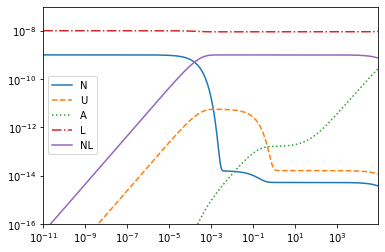We can even apply the transformation our reduced systems (doing so by hand is excessively painful and error prone):

In :
native_tLN = NativeCvodeSys.from_other(tsysLN)
_, _, info_tLN = integrate_and_plot(native_tLN, first_step=1e-9, nsteps=18000, atol=1e-9, rtol=1e-9)
{k: info_tLN[k] for k in ('nfev', 'njev', 'n_steps')}
INFO:pyodesys.native._base:In "/tmp/tmp6dehvnpj_pycodeexport_pyodesys_NativeCvodeCode", executing:
"/usr/bin/g++ -c -std=c++11 -Wall -Wextra -fPIC -O2 -ffast-math -funroll-loops -fopenmp -o ./odesys_anyode.o -DPYCVODES_NO_KLU=1 -DPYCVODES_NO_LAPACK=0 -DANYODE_NO_LAPACK=0 -I/usr/local/lib/python3.6/dist-packages/numpy/core/include -I/drone/src/github.com/bjodah/chempy/ci_cache/pyusrb/lib/python3.6/site-packages/pyodesys/native/sources -I/drone/src/github.com/bjodah/chempy/ci_cache/pyusrb/lib/python3.6/site-packages/pycvodes/include odesys_anyode.cpp"
INFO:pyodesys.native._base:In "/tmp/tmp6dehvnpj_pycodeexport_pyodesys_NativeCvodeCode", executing:
"/usr/bin/g++ -pthread -shared -std=c++11 -Wall -Wextra -fPIC -O2 -ffast-math -funroll-loops -fopenmp -o /tmp/tmp6dehvnpj_pycodeexport_pyodesys_NativeCvodeCode/_cvode_wrapper.cpython-36m-x86_64-linux-gnu.so -DPYCVODES_NO_KLU=1 -DPYCVODES_NO_LAPACK=0 -DANYODE_NO_LAPACK=0 -I/usr/local/lib/python3.6/dist-packages/numpy/core/include -I/drone/src/github.com/bjodah/chempy/ci_cache/pyusrb/lib/python3.6/site-packages/pyodesys/native/sources -I/drone/src/github.com/bjodah/chempy/ci_cache/pyusrb/lib/python3.6/site-packages/pycvodes/include odesys_anyode.o _cvode_wrapper.o -lsundials_nvecserial -lsundials_cvodes -lsundials_sunlinsollapackdense -lsundials_sunlinsollapackband -lblas -llapack -lpython3.6m"
time_wall = 0.0107, time_cpu = 0.0107, time_python = 2.84
Out:
{'nfev': 2896, 'njev': 46, 'n_steps': 1673}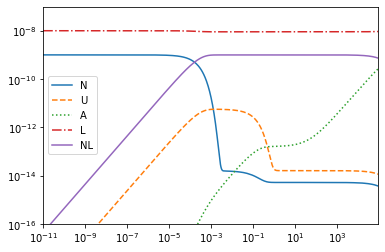In :
_, _, info_tLN = integrate_and_plot(tsysLN, first_step=1e-9, nsteps=18000, atol=1e-8, rtol=1e-8)
{k: info_tLN[k] for k in ('nfev', 'njev', 'n_steps')}
time_wall = 2.97, time_cpu = 2.97, time_python = 2.97
Out:
{'nfev': 2156, 'njev': 51, 'n_steps': 1249}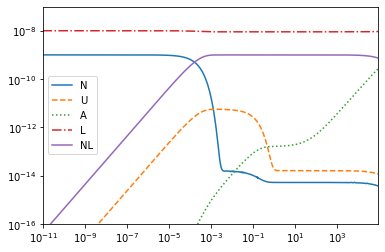In :
_, _, info_tLA = integrate_and_plot(tsysLA, first_step=0.0)
{k: info_tLA[k] for k in ('nfev', 'njev', 'n_steps')}
time_wall = 4.72, time_cpu = 4.72, time_python = 4.73
Out:
{'nfev': 3654, 'njev': 55, 'n_steps': 2474}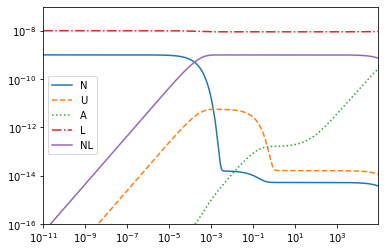Finally, let's take a look at the C++ code which was generated for us:

In :
print(open(next(filter(lambda s: s.endswith('.cpp'), native2._native._written_files))).read())
// -*- mode: read-only -*-
// This file was generated using pyodesys-0.12.5 at 2019-10-07T00:46:06.662936
// -*- coding: utf-8 -*-

// User provided system description: None
// Names of dependent variables: ('N', 'U', 'A', 'L', 'NL')
// Names of parameters: ('k_B', 'h', 'temperature', 'R', 'Ha_as', 'Sa_as', 'He_dis', 'Se_dis', 'Cp_dis', 'Tref_dis', 'Ha_f', 'Sa_f', 'He_u', 'Se_u', 'Cp_u', 'Tref_u', 'Ha_agg', 'Sa_agg')

#include <algorithm>
#include <iostream>
#include <limits>
#include <cmath>
#include <vector>
#include "odesys_anyode_iterative.hpp"
#include <type_traits>
#include <vector>

namespace {  // anonymous namespace for user-defined helper functions
std::vector<std::string> p_odesys_names = {"N", "U", "A", "L", "NL"};

template <typename T>
constexpr T vecmin(T&& a){
return std::forward<T>(a);
}
template <typename T1, typename T2>
constexpr typename std::common_type<T1, T2>::type vecmin(T1&& a, T2&& b){
return (a < b) ? std::forward<T1>(a) : std::forward<T2>(b);
}
template <typename T, typename... Ts>
constexpr typename std::common_type<T, Ts...>::type vecmin(T&& a, Ts&&... args){
return vecmin(std::forward<T>(a), vecmin(std::forward<Ts>(args)...));
}
std::vector<double> upper_conc_bounds(const double * const y){
auto bounds = std::vector<double>(5);
double cc;
cc = y + y;
cc = y + y + y + y;
bounds = vecmin(cc);
bounds = vecmin(cc);
bounds = vecmin(cc);
bounds = vecmin(cc);
bounds = vecmin(cc, cc);
return bounds;
}

}

using namespace std;

typedef double realtype;
typedef int32_t indextype;

namespace odesys_anyode {
template <>
struct OdeSys<realtype, indextype>: public AnyODE::OdeSysIterativeBase<realtype, indextype> {
std::vector<realtype> m_p;
std::vector<realtype> m_p_cse;
std::vector<realtype> m_atol;
std::vector<realtype> m_upper_bounds;
std::vector<realtype> m_lower_bounds;
std::vector<realtype> m_invar0;
realtype m_rtol;
realtype m_get_dx_max_factor;
bool m_error_outside_bounds;
realtype m_max_invariant_violation;
std::vector<realtype> m_special_settings;
OdeSys(const realtype * const,
std::vector<realtype>,
realtype,
realtype,
bool, realtype,
std::vector<realtype>);
int nrev=0;  // number of calls to roots
indextype get_ny() const override;
indextype get_nnz() const override;
int get_nquads() const override;
int get_nroots() const override;
realtype get_dx0(realtype, const realtype * const) override;
realtype get_dx_max(realtype, const realtype * const) override;
realtype max_euler_step(realtype, const realtype * const);
AnyODE::Status rhs(realtype t,
const realtype * const __restrict__ y,
realtype * const __restrict__ f) override;
AnyODE::Status dense_jac_cmaj(realtype t,
const realtype * const __restrict__ y,
const realtype * const __restrict__ fy,
realtype * const __restrict__ jac,
long int ldim,
realtype * const __restrict__ dfdt=nullptr) override;
AnyODE::Status dense_jac_rmaj(realtype t,
const realtype * const __restrict__ y,
const realtype * const __restrict__ fy,
realtype * const __restrict__ jac,
long int ldim,
realtype * const __restrict__ dfdt=nullptr) override;
AnyODE::Status sparse_jac_csc(realtype t,
const realtype * const __restrict__ y,
const realtype * const __restrict__ fy,
realtype * const __restrict__ data,
indextype * const __restrict__ colptrs,
indextype * const __restrict__ rowvals) override;
AnyODE::Status jtimes(const realtype * const __restrict__ vec,
realtype * const __restrict__ out,
realtype t,
const realtype * const __restrict__ y,
const realtype * const __restrict__ fy) override;
AnyODE::Status roots(realtype t, const realtype * const y, realtype * const out) override;
};

OdeSys<realtype, indextype>::OdeSys(const realtype * const params,
std::vector<realtype> atol,
realtype rtol,
realtype get_dx_max_factor,
bool error_outside_bounds,
realtype max_invariant_violation,
std::vector<realtype> special_settings) :
m_p_cse(5), m_atol(atol), m_rtol(rtol), m_get_dx_max_factor(get_dx_max_factor),
m_error_outside_bounds(error_outside_bounds), m_max_invariant_violation(max_invariant_violation),
m_special_settings(special_settings) {
m_p.assign(params, params + 23);

const auto cse_temporary0 = 1/(m_p*m_p);
const auto cse_temporary1 = m_p*m_p/m_p;
const auto cse_temporary2 = 1.0*cse_temporary1;
m_p_cse = cse_temporary2*exp(cse_temporary0*(-m_p + m_p*m_p));
m_p_cse = m_p_cse*exp(cse_temporary0*(-m_p - m_p*(-m_p + m_p) + m_p*(m_p + m_p*log(m_p/m_p))));
const auto cse_temporary5 = exp(cse_temporary0*(m_p*m_p - m_p));
m_p_cse = cse_temporary1*cse_temporary5;
m_p_cse = cse_temporary2*cse_temporary5*exp(cse_temporary0*(m_p*(m_p + m_p*log(m_p/m_p)) - m_p - m_p*(m_p - m_p)));
m_p_cse = cse_temporary2*exp(cse_temporary0*(-m_p + m_p*m_p));
use_get_dx_max = (m_get_dx_max_factor > 0.0) ? true : false;
if (m_max_invariant_violation != 0.0){

const realtype * const y = params + 18;
const auto cse0 = 1.0000000000000001e-9*y;
m_invar0.push_back(cse0 + 1.0000000000000001e-9*y);
m_invar0.push_back(cse0 + 1.0000000000000001e-9*y + 1.0000000000000001e-9*y + 1.0000000000000001e-9*y);
}

}

indextype OdeSys<realtype, indextype>::get_ny() const {
return 5;
}

indextype OdeSys<realtype, indextype>::get_nnz() const {
return -1;
}

int OdeSys<realtype, indextype>::get_nquads() const {
return 0;  // Not implemeneted yet (cvodes from Sundials supports this)
}

int OdeSys<realtype, indextype>::get_nroots() const {
return 0;
}

AnyODE::Status OdeSys<realtype, indextype>::rhs(realtype x,
const realtype * const __restrict__ y,
realtype * const __restrict__ f) {
AnyODE::ignore(x);
const auto cse0 = m_p_cse*y;
const auto cse1 = m_p_cse*y;
const auto cse2 = m_p_cse*y;
const auto cse3 = 1.0000000000000001e-9*m_p_cse*y*y;
const auto cse4 = cse2 - cse3;
const auto cse5 = m_p_cse*y;

f = cse0 - cse1 + cse4;
f = -cse0 + cse1 - cse5;
f = cse5;
f = cse4;
f = -cse2 + cse3;
this->nfev++;
if (m_error_outside_bounds){
if (m_lower_bounds.size() > 0) {
for (int i=0; i < 5; ++i) {
if (y[i] < m_lower_bounds[i]) {
std::cerr << "Lower bound (" << m_lower_bounds << ") for "
<< (p_odesys_names.size() ? p_odesys_names[i] : std::to_string(i))
<< " exceeded (" << y[i] << ") at x="<< x << "\n";
return AnyODE::Status::recoverable_error;
}
}
}
if (m_upper_bounds.size() > 0) {
for (int i=0; i < 5; ++i) {
if (y[i] > m_upper_bounds[i]) {
std::cerr << "Upper bound (" << m_upper_bounds << ") for "
<< (p_odesys_names.size() ? p_odesys_names[i] : std::to_string(i))
<< " exceeded (" << y[i] << ") at x="<< x << "\n";
return AnyODE::Status::recoverable_error;
}
}
}
}
if (m_max_invariant_violation != 0.0){
const auto cse0 = 1.0000000000000001e-9*y;
if (std::abs(cse0 + 1.0000000000000001e-9*y - m_invar0) > ((m_max_invariant_violation > 0) ? m_max_invariant_violation : std::abs(m_max_invariant_violation*m_invar0) - m_max_invariant_violation)) {
std::cerr << "Invariant (0) violation at x=" << x << "\n";
return AnyODE::Status::recoverable_error;
}
if (std::abs(cse0 + 1.0000000000000001e-9*y + 1.0000000000000001e-9*y + 1.0000000000000001e-9*y - m_invar0) > ((m_max_invariant_violation > 0) ? m_max_invariant_violation : std::abs(m_max_invariant_violation*m_invar0) - m_max_invariant_violation)) {
std::cerr << "Invariant (1) violation at x=" << x << "\n";
return AnyODE::Status::recoverable_error;
}
}
return AnyODE::Status::success;
}

AnyODE::Status OdeSys<realtype, indextype>::jtimes(
const realtype * const __restrict__ v,
realtype * const __restrict__ Jv,
realtype x,
const realtype * const __restrict__ y,
const realtype * const __restrict__ fy) {
AnyODE::ignore(v); AnyODE::ignore(Jv); AnyODE::ignore(x);
AnyODE::ignore(y); AnyODE::ignore(fy);
return AnyODE::Status::unrecoverable_error;
}

AnyODE::Status OdeSys<realtype, indextype>::dense_jac_cmaj(realtype x,
const realtype * const __restrict__ y,
const realtype * const __restrict__ fy,
realtype * const __restrict__ jac,
long int ldim,
realtype * const __restrict__ dfdt) {
// The AnyODE::ignore(...) calls below are used to generate code free from false compiler warnings.
AnyODE::ignore(fy);  // Currently we are not using fy (could be done through extensive pattern matching)
AnyODE::ignore(x);

const auto cse0 = 1.0000000000000001e-9*m_p_cse;
const auto cse1 = cse0*y;
const auto cse2 = -cse1;
const auto cse3 = cse0*y;
const auto cse4 = -cse3;

jac[ldim*0 + 0] = cse2 - m_p_cse;
jac[ldim*0 + 1] = m_p_cse;
jac[ldim*0 + 3] = cse2;
jac[ldim*0 + 4] = cse1;

jac[ldim*1 + 0] = m_p_cse;
jac[ldim*1 + 1] = -m_p_cse - m_p_cse;
jac[ldim*1 + 2] = m_p_cse;

jac[ldim*3 + 0] = cse4;
jac[ldim*3 + 3] = cse4;
jac[ldim*3 + 4] = cse3;

jac[ldim*4 + 0] = m_p_cse;
jac[ldim*4 + 3] = m_p_cse;
jac[ldim*4 + 4] = -m_p_cse;

if (dfdt){
dfdt = 0;
dfdt = 0;
dfdt = 0;
dfdt = 0;
dfdt = 0;
}
this->njev++;
return AnyODE::Status::success;
}
AnyODE::Status OdeSys<realtype, indextype>::dense_jac_rmaj(realtype x,
const realtype * const __restrict__ y,
const realtype * const __restrict__ fy,
realtype * const __restrict__ jac,
long int ldim,
realtype * const __restrict__ dfdt) {
// The AnyODE::ignore(...) calls below are used to generate code free from false compiler warnings.
AnyODE::ignore(fy);  // Currently we are not using fy (could be done through extensive pattern matching)
AnyODE::ignore(x);

const auto cse0 = 1.0000000000000001e-9*m_p_cse;
const auto cse1 = cse0*y;
const auto cse2 = -cse1;
const auto cse3 = cse0*y;
const auto cse4 = -cse3;

jac[ldim*0 + 0] = cse2 - m_p_cse;
jac[ldim*0 + 1] = m_p_cse;
jac[ldim*0 + 3] = cse4;
jac[ldim*0 + 4] = m_p_cse;

jac[ldim*1 + 0] = m_p_cse;
jac[ldim*1 + 1] = -m_p_cse - m_p_cse;

jac[ldim*2 + 1] = m_p_cse;

jac[ldim*3 + 0] = cse2;
jac[ldim*3 + 3] = cse4;
jac[ldim*3 + 4] = m_p_cse;

jac[ldim*4 + 0] = cse1;
jac[ldim*4 + 3] = cse3;
jac[ldim*4 + 4] = -m_p_cse;

if (dfdt){
dfdt = 0;
dfdt = 0;
dfdt = 0;
dfdt = 0;
dfdt = 0;
}
this->njev++;
return AnyODE::Status::success;
}

realtype OdeSys<realtype, indextype>::get_dx0(realtype x, const realtype * const y) {

m_upper_bounds = upper_conc_bounds(y);
m_lower_bounds.resize(5);
return m_rtol*std::min(get_dx_max(x, y), 1.0);

}

AnyODE::Status OdeSys<realtype, indextype>::sparse_jac_csc(realtype x,
const realtype * const __restrict__ y,
const realtype * const __restrict__ fy,
realtype * const __restrict__ data,
indextype * const __restrict__ colptrs,
indextype * const __restrict__ rowvals) {
AnyODE::ignore(x); AnyODE::ignore(y); AnyODE::ignore(fy);
AnyODE::ignore(data); AnyODE::ignore(colptrs); AnyODE::ignore(rowvals);
return AnyODE::Status::unrecoverable_error;
}

realtype OdeSys<realtype, indextype>::get_dx_max(realtype x, const realtype * const y) {
auto fvec = std::vector<realtype>(5);
auto hvec = std::vector<realtype>(5);
rhs(x, y, &fvec);
for (indextype idx=0; idx < 5; ++idx){
if (fvec[idx] == 0) {
hvec[idx] = std::numeric_limits<realtype>::max();
} else if (fvec[idx] > 0) {
hvec[idx] = std::abs(m_upper_bounds[idx] - y[idx])/fvec[idx];
} else { // fvec[idx] < 0
hvec[idx] = std::abs((m_lower_bounds[idx] - y[idx])/fvec[idx]);
}
}
const auto result = *std::min_element(std::begin(hvec), std::end(hvec));
if (m_get_dx_max_factor == 0.0)
return result;
else if (m_get_dx_max_factor < 0.0)
return -m_get_dx_max_factor*result;
else
return m_get_dx_max_factor*result;
}

AnyODE::Status OdeSys<realtype, indextype>::roots(realtype x, const realtype * const y, realtype * const out) {
AnyODE::ignore(x); AnyODE::ignore(y); AnyODE::ignore(out);
return AnyODE::Status::success;
}
}